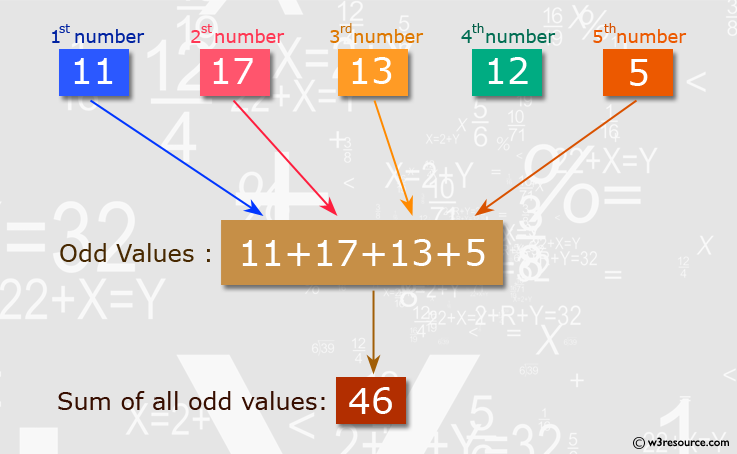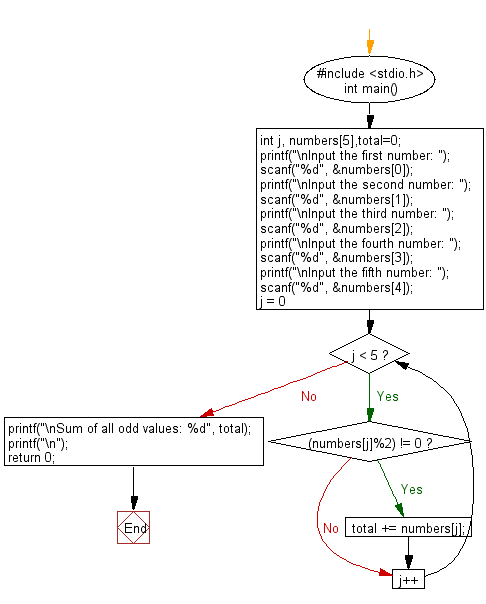﻿ C : Read 5 numbers and sum of all odd values between them

# C Exercises: Read 5 numbers and sum of all odd values between them

## C Basic Declarations and Expressions: Exercise-22 with Solution

Write a C program that reads 5 numbers and sums all odd values between them.

Pictorial Presentation:C Code:

``````#include <stdio.h>
int main() {
int j, numbers, total=0; // Declare variables for loop counter, an array of numbers, and total

// Prompt user for five numbers and store them in the array
printf("\nInput the first number: ");
scanf("%d", &numbers);
printf("\nInput the second number: ");
scanf("%d", &numbers);
printf("\nInput the third number: ");
scanf("%d", &numbers);
printf("\nInput the fourth number: ");
scanf("%d", &numbers);
printf("\nInput the fifth number: ");
scanf("%d", &numbers);

// Loop through the numbers to find and sum the odd ones
for(j = 0; j < 5; j++) {
if((numbers[j]%2) != 0)
{
total += numbers[j];
}
}

// Print the sum of odd values
printf("\nSum of all odd values: %d", total);
printf("\n");

return 0;
}
``````

Sample Output:

```Input the first number: 11

Input the second number: 17

Input the third number: 13

Input the fourth number: 12

Input the fifth number: 5

Sum of all odd values: 46
```

Flowchart:C Programming Code Editor:

What is the difficulty level of this exercise?

Test your Programming skills with w3resource's quiz.

﻿